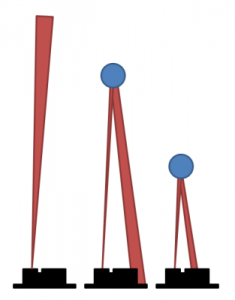# GP2D12 Project

In this small project we are going to use Sharp GP2D12 sensor and an Arduino Uno to measure the distance between the sensor and an object. You can use this to follow a wall for example. With use of a servo you can provide a wide ‘view’ by pointing the sensor to the left and right (and anything in between). This can be used to let a robot ‘see’ it’s surroundings and calculate a path to follow.The Sharp GP2D12 is a popular sensor for Robot builders. The sensor sends out a beam of light and uses triangulation to measure the distance. The detection range of the GP2D12 is between 10 cm (4 inch) and 80 cm (32 inch). The beam is 6 cm wide at 80 cm distance, so keep this in mind when using this sensor.In the image above you can see that if anything is in the way of the beam the sensor will receive a reflection of the light. The use of the GP2D12 with an Arduino is simple. We have to apply power and connect the output with an analog port of the Arduino.To calculate the values from the analog port to a distance in cm a formula is needed. I found a extensive explanation on the Acroname website: http://www.acroname.com/robotics/info/articles/irlinear/irlinear.html

The formula is incorporated into the following code:

```// Arduino Code to measure distance with a Sharp GP2D12 sensor
// www.swanrobotics.com

int IR_SENSOR = 0; // Sensor is connected to the analog A0
int intSensorResult = 0; //Sensor result
float fltSensorCalc = 0; //Calculated value

void setup()
{
Serial.begin(9600); // Setup communication with computer to present results serial monitor
}

void loop()
{
// read the value from the ir sensor

intSensorResult = analogRead(IR_SENSOR); //Get sensor value
fltSensorCalc = (6787.0 / (intSensorResult - 3.0)) - 4.0; //Calculate distance in cm

Serial.print(fltSensorCalc); //Send distance to computer
Serial.println(" cm"); //Add cm to result
delay(200); //Wait
}
```

When executing the code on your Arduino and watch the serial monitor you should see something like this: